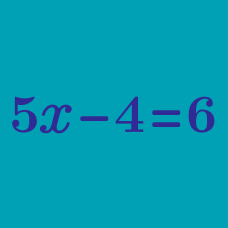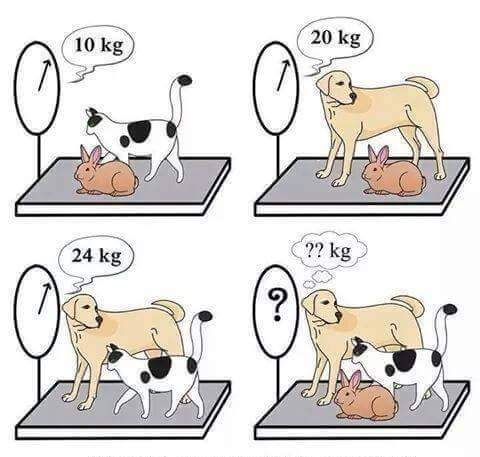Algebra

# Solving Equations: Level 1 ChallengesFind the total weight of the three animals.

$\large \begin{cases} {x^4 + 5y = 6 } \\ {x^2 y^2 + 5x = 6 } \end{cases}$

Solve the system of equations above.

$\large x + y = 55 \\ \large x - y = 27$

If $x$ and $y$ satisfy the equations above, find the value of the larger variable.A frog is at the bottom of a 78 meter well. Each day he leaps 4 meters up the well. At night, while he is asleep, he slips 2 meters backwards.

How many days does it take him to escape from the well?

Note: The frog escapes from the well once he climbs up a total of 78 meters.×

Problem Loading...

Note Loading...

Set Loading...